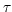GVD_cT_Gate
Subsections

## GVD_cT_Gate

Vh (Volt) :
Inflection point of the sigmoidal function p(V)
Vc (Volt) :
Slope of the sigmoidal function p(V)
Ts :
Voltage independent time constantnummethod (flag) :
Numerical method for the solution of the differential equation: Exp. Euler = 0, Crank-Nicolson = 1
k (1) :
The exponent of the gate
p :
The state variable.